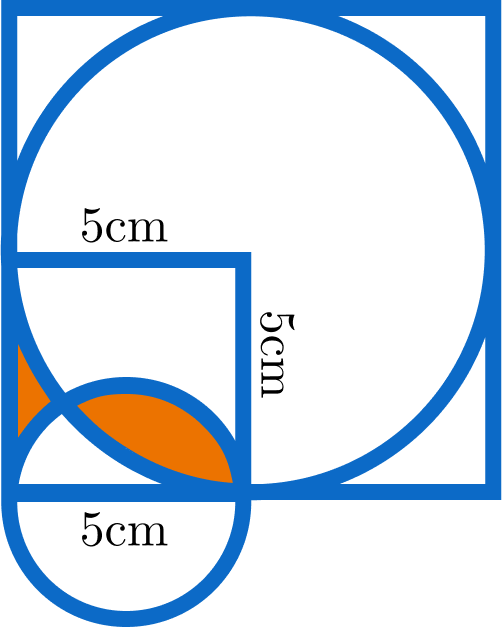# Sweet Red FishThe figure above shows that a large circle with radius $5\text{ cm}$ is inscribed in a square and another circle with diameter $5\text{ cm}$ is only half inside that square.

Calculate the area of the shaded region (colored red) in the figure. Give your answer in $\text{cm}^2$ to three decimal places.

Details and Assumptions:

• You may use the approximation $\pi = 3.14159$ and $\sin^{-1} \left ( \frac 4 5 \right ) = 0.92729$.
×